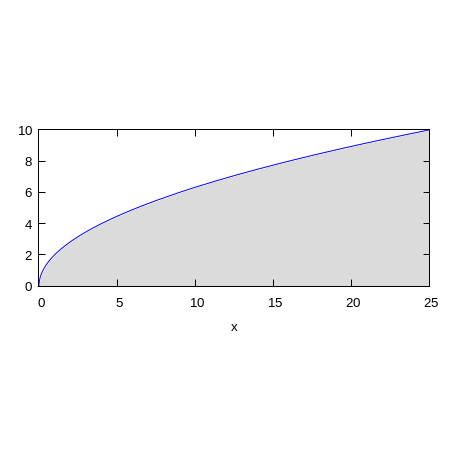# Consider the integral integral_0^{25} integral_0^{2 square root x} f (x,y) dydx. Sketch the...

## Question:

Consider the integral

{eq}I=\int_0^{25} \int_0^{2 \sqrt x} f (x,y) \;dy\,dx {/eq}. Sketch the region of integration and change the order of integration.

{eq}\int_a^b \int_{g_1(y)}^{g_2(y)} f(x,y)\;dx\,dy {/eq}

{eq}a=?\qquad b=?\qquad g_1(y)=?\qquad g_2(y)=? {/eq}

## Iterated Integrals; Changing Order of Integration:

We want to change the order of integration, so as to integrate first with respect to the {eq}x {/eq} variable rather than with respect to {eq}y {/eq} as it is written in the problem statement. However, we wont evaluate the integral because the integrand {eq}f(x,y) {/eq} is not known (except that it is integrable in the indicated region).

## Answer and Explanation:

The diagram below presents the region of integration and the blue curve is the portion of the {eq}y=2\sqrt{x} {/eq} for {eq}x\in[0,25] {/eq}.From the equation {eq}y=2\sqrt{x} {/eq} we can solve for {eq}x {/eq} and we get {eq}x=\left(\frac{y}{2}\right)^2=\frac{y^2}{4}. {/eq} We notice that {eq}y {/eq} is in the range {eq}0\le y\le 10=2\sqrt{25}. {/eq}

The region of integration can be described as follows: For any {eq}y\in[0,10] {/eq} we have {eq}x\in\left [\frac{y^2}{4},25\right ]. {/eq} Now we can change the order of integration:

{eq}\begin{align*} I&=\int_0^{25} \int_0^{2 \sqrt x} f (x,y) \;dy\,dx&[\text{change order of integration} ]\\ I&=\int_{0}^{10}\int_{\frac{y^2}{4}}^{25}f(x,y)\,dx\,dy. \end{align*} {/eq}

So we can write:

{eq}\boxed{I=\int_a^b \int_{g_1(y)}^{g_2(y)} f(x,y)\;dx\,dy= \int_{0}^{10}\int_{\frac{y^2}{4}}^{25}f(x,y)\,dx\,dy}, {/eq}

so,

{eq}a=0,\qquad b=10,\qquad g_1(y)=\dfrac{y^2}{4}, \qquad g_2(y)=25. {/eq}

#### Learn more about this topic:Double Integration: Method, Formulas & Examples

from AP Calculus AB & BC: Help and Review

Chapter 12 / Lesson 15
33K